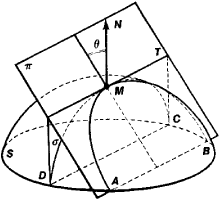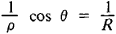# Meusnier's Theorem

Also found in: Wikipedia.

## Meusnier's theorem

[mən′yāz ‚thir·əm]
(mathematics)
A theorem stating that the curvature of a surface curve equals the curvature of the normal section through the tangent to the curve divided by the cosine of the angle between the plane of this normal section and the osculating plane of the curve.

## Meusnier’s Theorem

a theorem in differential geometry that establishes the property of curvatures of plane sections of a surface. Let TT be an arbitrary plane through the tangent MT at the point M to the surface S (see Figure 1), θ be the angle thatFigure 1

the plane makes with the normal MN to the surface, and 1/R be the curvature at M of the curve DMC along which the surface S is intersected by the plane cr passing through the normal MN and the line MT (DMC is called the normal section of the surface). Then the curvature 1/ρ at M of the curve AMB along which the surface S is intersected by the plane π is related to the curvature 1/R of the normal section byThis formula expresses Meusnier’s theorem. Meusnier’s theorem was established by J. Meusnier in 1776 but published only in 1785.

### REFERENCE

Rashevskii, P. K. Kurs differentiarnoi geometrii, 4th ed. Moscow, 1956.
Site: Follow: Share:
Open / Close